# How to Calculate and Solve for the Height and Volume of a Conical Frustum | The Calculator Encyclopedia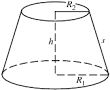The image above is a conical frustum.

To compute the volume of a conical frustum, three essential parameters are needed and this parameters are radius of the lower base (R), radius of the upper base (r) and height (h).

The formula for calculating the volume of a conical frustum:

V = πh(R² + Rr + r²)3

Where;
V = Volume of the conical frustum
R = Radius of the lower base
r = Radius of the upper base
h = Height of the conical frustum

Let’s solve an example;
Find the volume of the conical frustum when the lower base is 5 cm with an upper base of 9 cm and a height of 11 cm.

This implies that;
R = Radius of the lower base = 5 cm
r = Radius of the upper base = 9 cm
h = Height of the conical frustum = 11 cm

V = π(11)((5)² + (5)(9) + (9)²)3
V = (34.557)((25) + (45) + (81))3
V = (34.557)(151)3
V = 5218.1853
V = 1739.39

Therefore, the volume of the conical frustum is 1739.39 cm3.

Nickzom Calculator – The Calculator Encyclopedia is capable of calculating the volume of a conical frustum.

To get the answer and workings of the volume of a conical frustum using the Nickzom Calculator – The Calculator Encyclopedia. First, you need to obtain the app.

You can get this app via any of these means:

To get access to the professional version via web, you need to register and subscribe for NGN 1,500 per annum to have utter access to all functionalities.
You can also try the demo version via https://www.nickzom.org/calculator

Once, you have obtained the calculator encyclopedia app, proceed to the Calculator Map, then click on Mensuration under the Mathematics section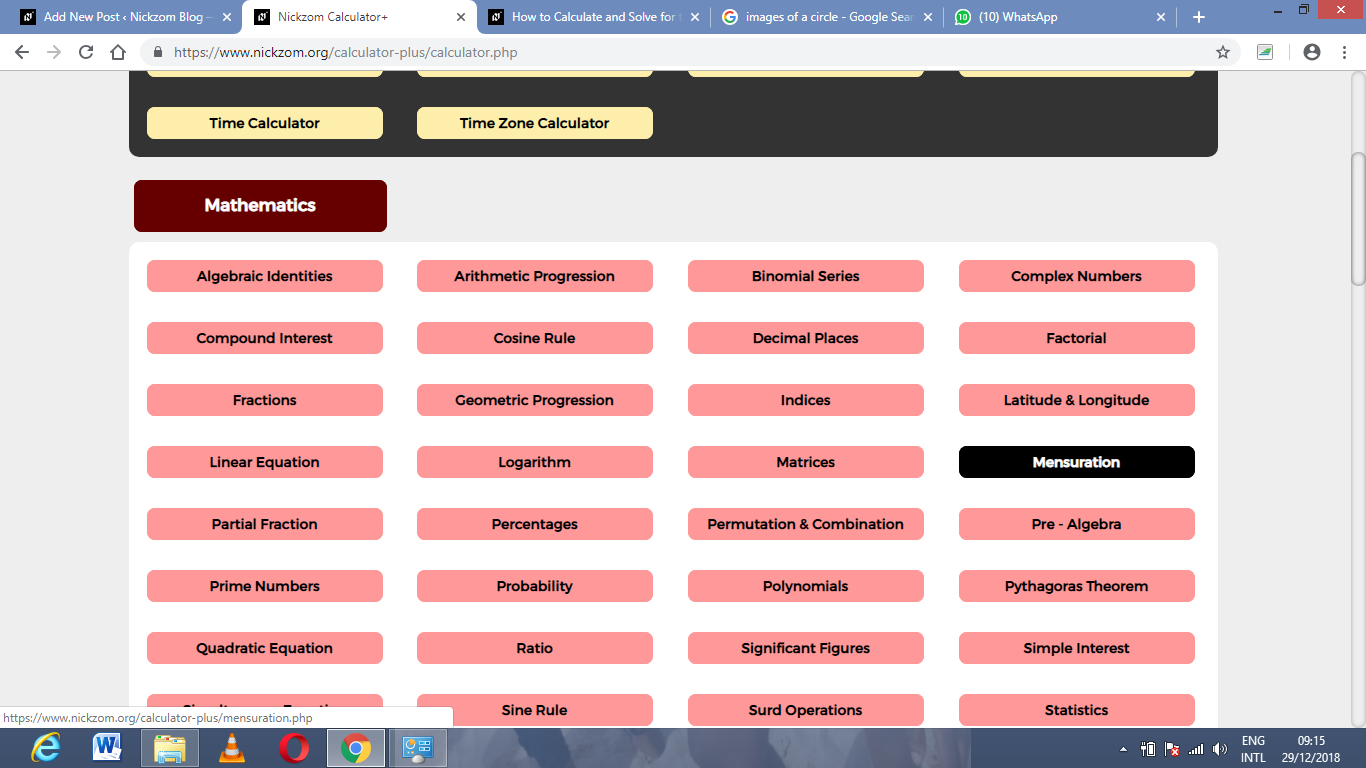Now, click on Volume of a conical frustum under Mensuration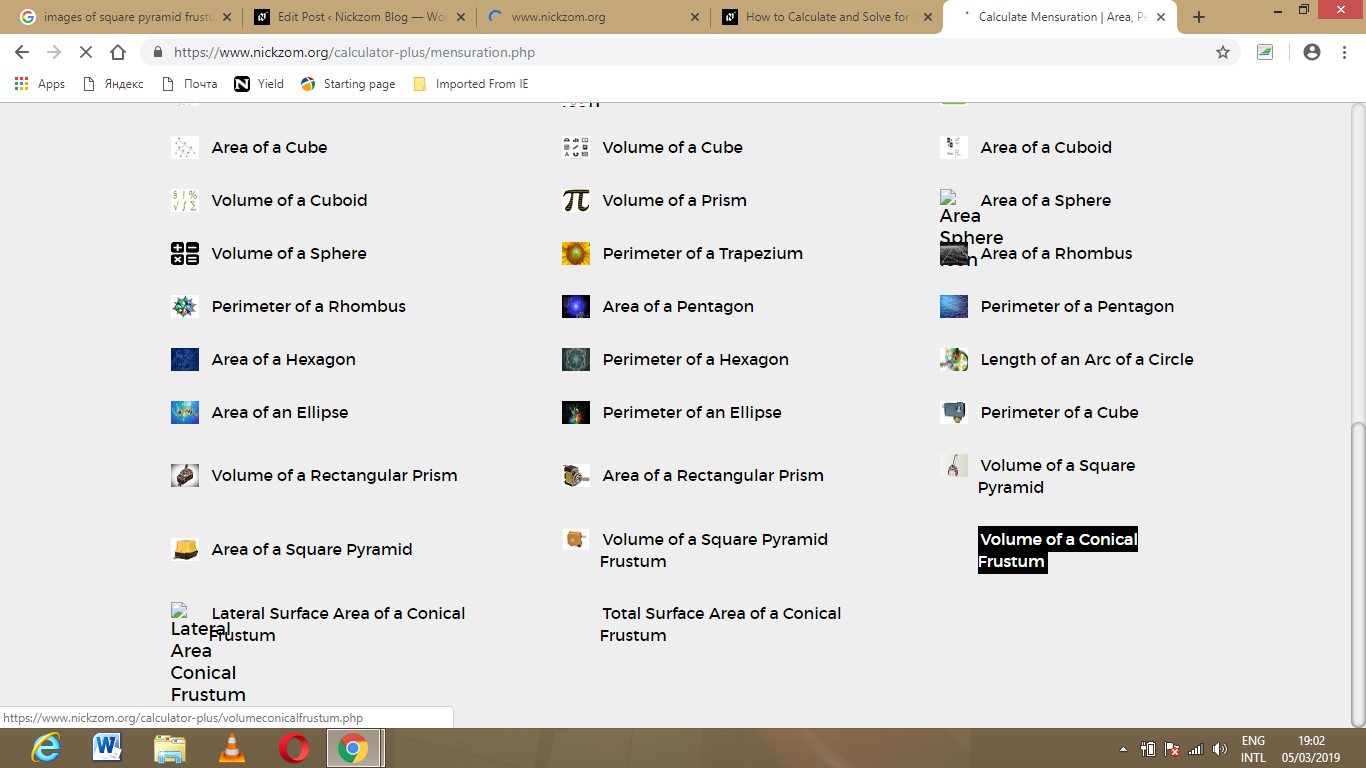The screenshot below displays the page or activity to enter your values, to get the answer for the volume of a conical frustum according to the respective parameters which are the radius of the lower base (R), radius of the upper base (r) and height (h).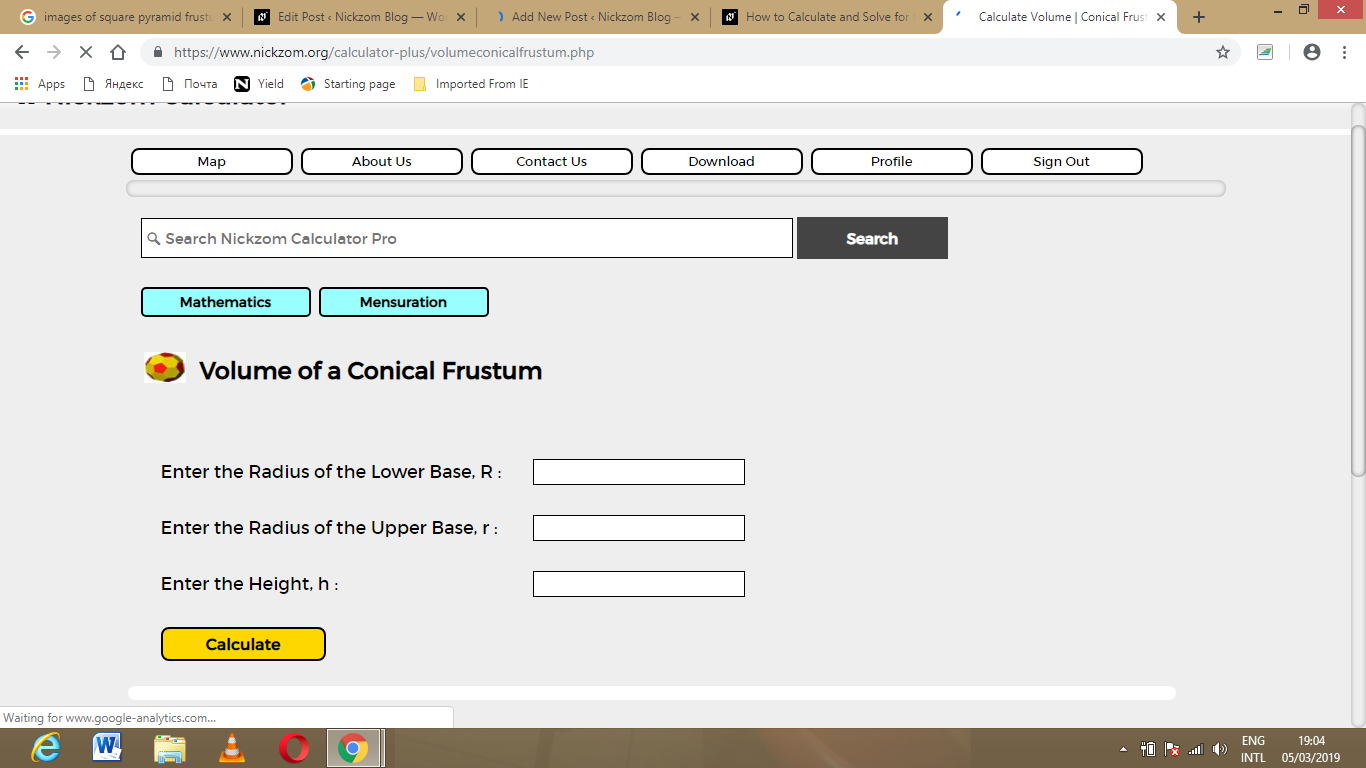Now, enter the values appropriately and accordingly for the parameters as required by the example above where the radius of the lower base (R) is 5 cm, radius of the upper base (r) is 9 cm and height (h) is 11 cm.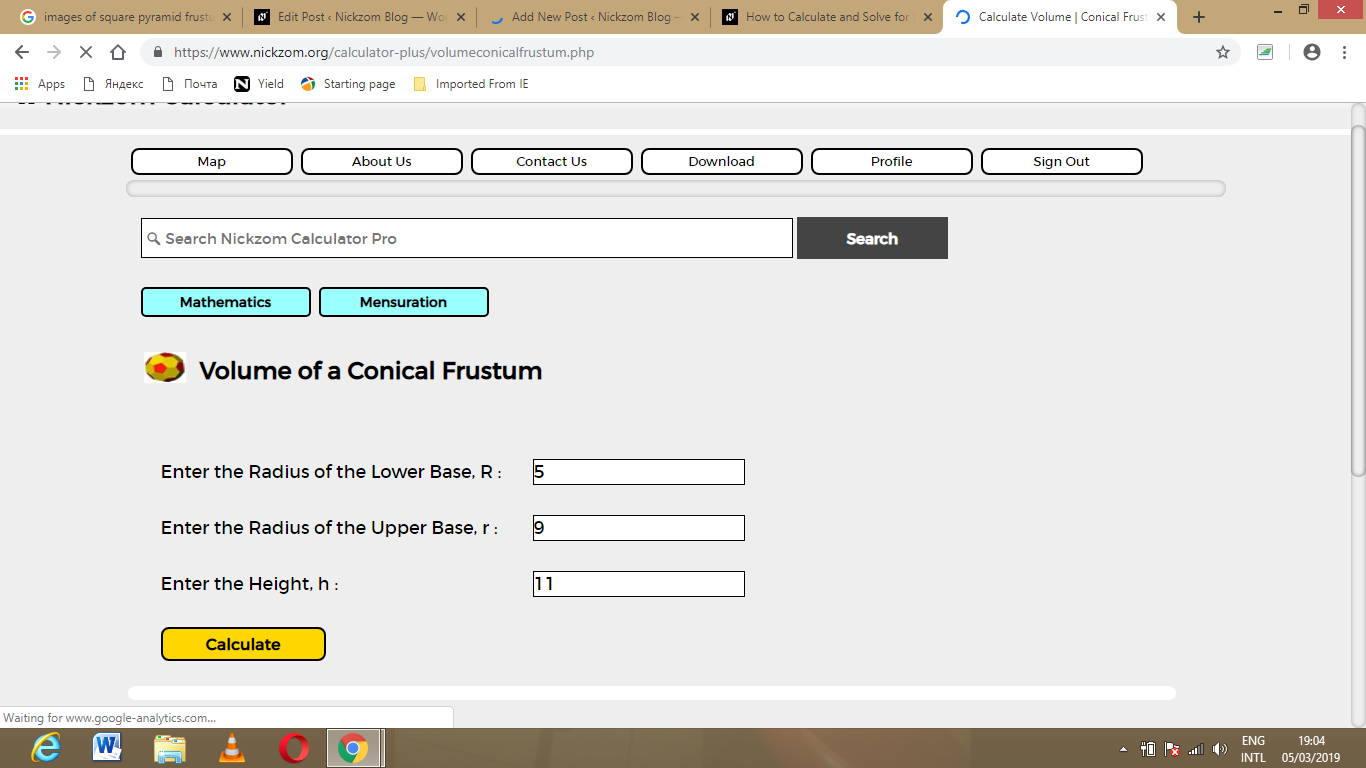Finally, Click on Calculate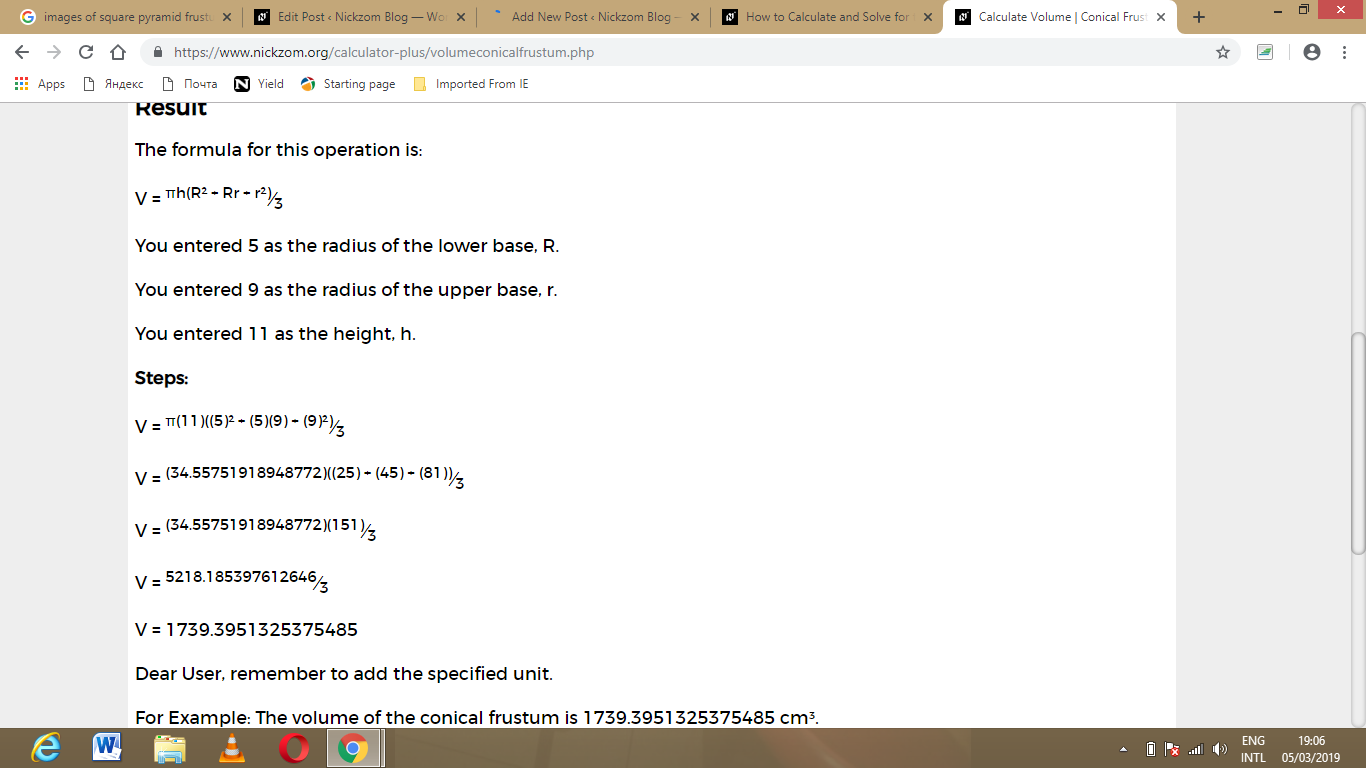As you can see from the screenshot above, Nickzom Calculator – The Calculator Encyclopedia solves for the volume of a conical frustum and presents the formula, workings and steps too.